# Cancer Diagnosis — Stochastic Gradient Descent Algorithms

In my earlier post regarding Logistic-regression loss minimization we had seen that by changing the form of the loss function we can derive other machine learning models. It’s precisely this that we are going to talk about in this post.

Below is a table that shows a few loss functions and their associated machine learning algorithms.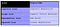Mapping Los to M/L Algorithms

To help us explore this concept further we will use the SGDClassifier provided by SKlearn

The path we will follow is:

• Gradient Descent
• Stochastic Gradient Descent
• Applying this to Logistic Regression
• SkLearn’s SGDClassifier
• Logistic Regression implementation using SGDClassifier

Let’s consider Logistic loss :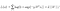Logistic Loss

In the above equation, x and y are cionstants , coz they belong D-train. Hence, the above equation depends on W, which is a vector.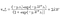Grad of L w.r.t W

Solving the above equation is hard , hence we use gradient descent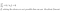Grad of L w.r.t W is zero

## Gradient Descent Algorithm

This is an iterative algorithm.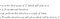SGD Algorithm basic

Slope changes it’s sign from +ve to -ve when slope = 0 at minima

Slope decreases/increases as we move towards minima.

At minima, slope sign changes from +ve to -ve.

As we closer to x* slope reduces or may increasewhen we move in the other diretion.

Let’s walkthru the pseudo-code for this algorithm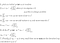Gradient Descent Algorithm pseudocode

## Learning Rate (eta)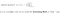Learning Rate introduction

Learning rate(eta) denoted by r is a constant . There can be a situation where the calculated x vaules oscillate around the minimum.

The remedy to this oscillating problem is to change learning rate in each iteration . We can think learning rate r as a function of i the iteration value of the loop of the Gradient descent algorithm.

We define a function which takes the learning rate as input and outputs a value that can be used as the step size or learning rate.

The function should be devised in such a way that the learning rate is reduced in small quantities for each iteration.

Gradient Descent for Linear Regression

In all above steps we are summing over all the n-points for every iteration.

Usually n- is large upto 1 million in real life Data Science projects and hence this calculation can be time consuming.

By using Stochastic Gradient Descent we will reduce the time consumed to solve the problem.

Stochastic Gradient Algorithm(SGD)

This is the most important optimization algorithm in Machine Learning.

The problem with Gradient Descent, is that for all iterations till we converge we are using all n-points.

In SGD, we pick a smaller set of k-points , where k is greater or equal to 1 but significantly less than n .

1 < k << n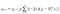summing over k points

If we perform enough iterations of the above we will get W*.

There is proof that Wgd* = Wsgd*, it is complex and hence I haven’t ventured into it .

For every iteration, the k-points we pick has to be different which is very important requirement for SGD.

Linear regression didn’t have any constraintsbut logistic regression has a constraint, hence let look at briefly what is constraint optimization.

Constraint Optimization

General constrainted optimization looks like

Logistic Regression viewed as Constraint Optimization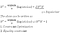Logistic Regression as Constrained Optimization formulation

The above is an high level overview of solving logisitc regression using constrainted optimization and can be used when you write your own classifier.Now, let’s see how Sklearn’s SGDClassifier has implented logistic regression using logloss and alpha as the hyper parameter.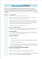Copyright Sklearn

The first parameter we will change is the ‘loss” parameter, to “log” to make the classifier solve the problem using logistic regression.

Will set parameter “penalty” to “l2” for l2 regularization.

The next parameter is “alpha” which is the multiplier term of the regularizer denoted by “lamda” in mathematical formulations. This is the hyper parameter which needs to get tuned.

The other parameter is “class_weight”, which is used in situations when the dataset is im-balanced. Our Cancer dataset is highly imbalanced hence we will use two process wherein we will set this value to “balanced” and then the default value of “None”.

The final parameter is “learning_rate” which we set as optimal.

## Logistic Regression using SGDClassifier Implementation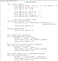Logistic Regression thru SGDClassifier

## Logistic Regression Calling Code — With Class_Weight = ‘Balanced’

Code to perform Hyper-parameter tuning

`cancdiag.sgdclf_cmn_code_hypertun(logregrbal,cancdiag.X_test_1hotCdg,cancdiag.x_train_1hotCdg,cancdiag.x_cval_1hotCdg,cancdiag.y_test,cancdiag.y_trn,cancdiag.y_cval)`

Code for testing the Logistic Regression Classifier

Hyper Parameter tuning output

Confusion , Precision and Recall matrices

With Class_Weight= ‘Default’

Hyper Parameter tuning output

## Inference and Conclusion

• Logistic Regression using the SGDClassifier is performing a shade better than Random Forest . In Random Forest number of mis-classified points was 36% whereas with Logistic Regression using the SGDClassifier with Class_Weight equal to Default is 36.5 %
• The test log loss for Random forest is 1.1291 where for Logistic Regression using the SGDClassifier with Class_Weight equal to Default is 1.111896
• Logistic Regression using the SGDClassifier with Class_Weight equal to Default is also able to atleast predict 1 data point belonging to class label 9 whereas Logistic Regression using the SGDClassifier with Class_Weight equal to Balanced came up with a blank

The code for this is available at

https://github.com/ariyurjana/Personalized_Cancer_diagnosis/blob/13c1ebab26b893910e4e8aed2dd9b94f3f2e22c0/PersCancDiag_logregr_sgd.ipynb

The PDF version is available at

https://github.com/ariyurjana/Personalized_Cancer_diagnosis/blob/13c1ebab26b893910e4e8aed2dd9b94f3f2e22c0/PersCancDiag_logregr_sgd.pdf

References

In the making Machine Learner programmer music lover

## More from Janardhanan a r

In the making Machine Learner programmer music lover